GFG App
Open AppBrowser
Continue

# C Arrays

Array in C is one of the most used data structures in C programming. It is a simple and fast way of storing multiple values under a single name. In this article, we will study the different aspects of array in C language such as array declaration, definition, initialization, types of arrays, array syntax, advantages and disadvantages, and many more.

## What is Array in C?

An array in C is a fixed-size collection of similar data items stored in contiguous memory locations. It can be used to store the collection of primitive data types such as int, char, float, etc., and also derived and user-defined data types such as pointers, structures, etc.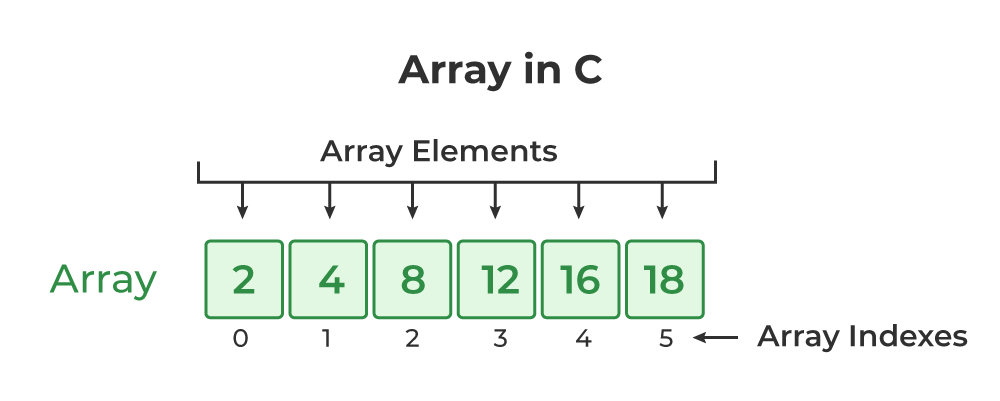## C Array Declaration

In C, we have to declare the array like any other variable before using it. We can declare an array by specifying its name, the type of its elements, and the size of its dimensions. When we declare an array in C, the compiler allocates the memory block of the specified size to the array name.

### Syntax of Array Declaration

```data_type array_name [size];
or
data_type array_name [size1] [size2]...[sizeN];```

where N is the number of dimensions.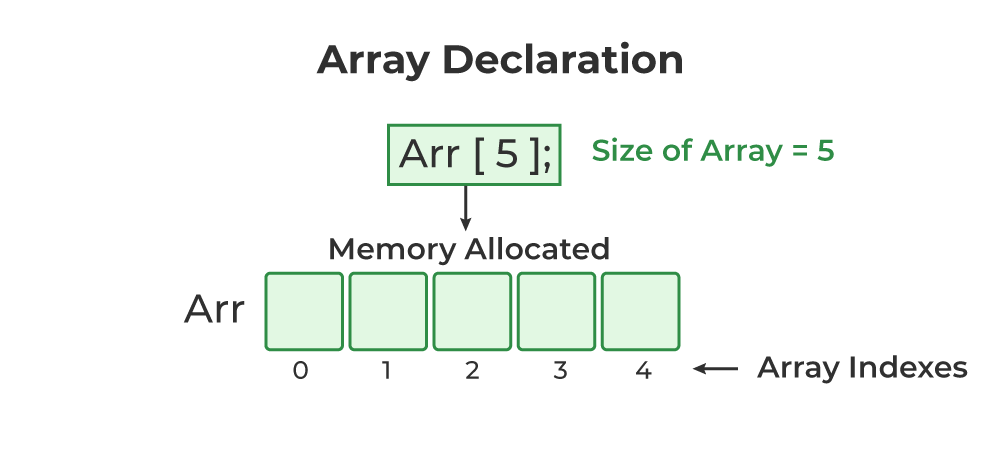The C arrays are static in nature, i.e., they are allocated memory at the compile time.

## C

 `// C Program to illustrate the array declaration` `#include `   `int` `main()` `{`   `    ``// declaring array of integers` `    ``int` `arr_int;` `    ``// declaring array of characters` `    ``char` `arr_char;`   `    ``return` `0;` `}`

## C Array Initialization

Initialization in C is the process to assign some initial value to the variable. When the array is declared or allocated memory, the elements of the array contain some garbage value. So, we need to initialize the array to some meaningful value. There are multiple ways in which we can initialize an array in C.

### 1. Array Initialization with Declaration

In this method, we initialize the array along with its declaration. We use an initializer list to initialize multiple elements of the array. An initializer list is the list of values enclosed within braces { } separated b a comma.

`data_type array_name [size] = {value1, value2, ... valueN};`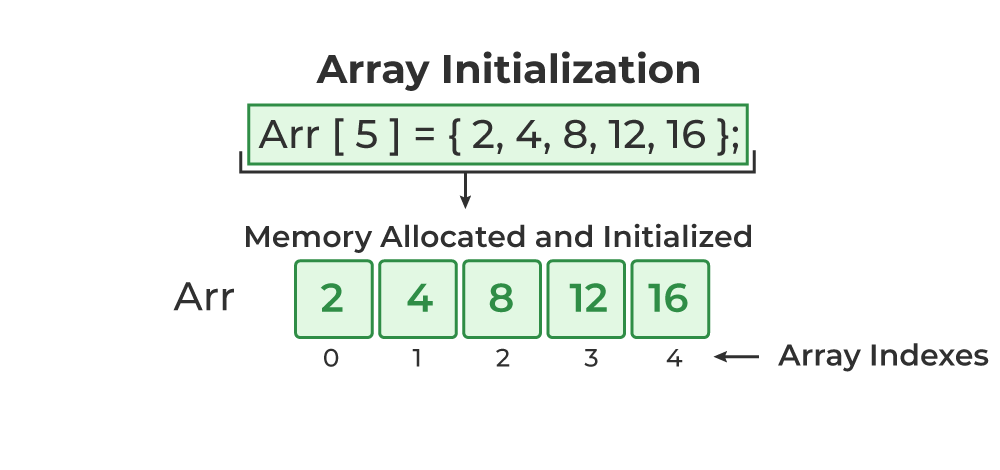### 2. Array Initialization with Declaration without Size

If we initialize an array using an initializer list, we can skip declaring the size of the array as the compiler can automatically deduce the size of the array in these cases. The size of the array in these cases is equal to the number of elements present in the initializer list as the compiler can automatically deduce the size of the array.

`data_type array_name[] = {1,2,3,4,5};`

The size of the above arrays is 5 which is automatically deduced by the compiler.

### 3. Array Initialization after Declaration (Using Loops)

We initialize the array after the declaration by assigning the initial value to each element individually. We can use for loop, while loop, or do-while loop to assign the value to each element of the array.

```for (int i = 0; i < N; i++) {
array_name[i] = valuei;
}```

## C

 `// C Program to demonstrate array initialization` `#include `   `int` `main()` `{`   `    ``// array initialization using initialier list` `    ``int` `arr = { 10, 20, 30, 40, 50 };`   `    ``// array initialization using initializer list without` `    ``// specifying size` `    ``int` `arr1[] = { 1, 2, 3, 4, 5 };`   `    ``// array initialization using for loop` `    ``float` `arr2;` `    ``for` `(``int` `i = 0; i < 5; i++) {` `        ``arr2[i] = (``float``)i * 2.1;` `    ``}` `    ``return` `0;` `}`

## Access Array Elements

We can access any element of an array in C using the array subscript operator [ ]  and the index value i of the element.

`array_name [index];`

One thing to note is that the indexing in the array always starts with 0, i.e., the first element is at index 0 and the last element is at N – 1 where N is the number of elements in the array.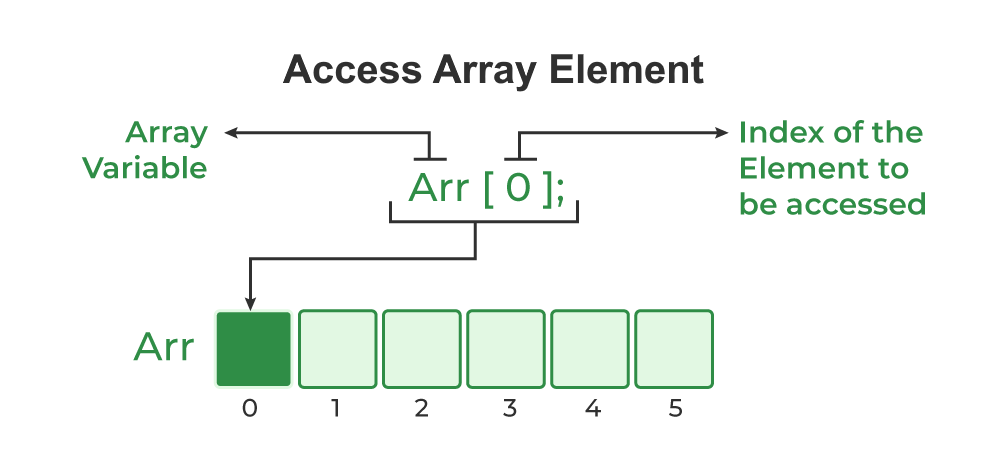## C

 `// C Program to illustrate element access using array` `// subscript` `#include `   `int` `main()` `{`   `    ``// array declaration and initialization` `    ``int` `arr = { 15, 25, 35, 45, 55 };`   `    ``// accessing element at index 2 i.e 3rd element` `    ``printf``(``"Element at arr: %d\n"``, arr);`   `    ``// accessing element at index 4 i.e last element` `    ``printf``(``"Element at arr: %d\n"``, arr);`   `    ``// accessing element at index 0 i.e first element` `    ``printf``(``"Element at arr: %d"``, arr);`   `    ``return` `0;` `}`

Output

```Element at arr: 35
Element at arr: 55
Element at arr: 15```

## Update Array Element

We can update the value of an element at the given index i in a similar way to accessing an element by using the array subscript operator [ ] and assignment operator =.

`array_name[i] = new_value;`

## C Array Traversal

Traversal is the process in which we visit every element of the data structure. For C array traversal, we use loops to iterate through each element of the array.

### Array Traversal using for Loop

```for (int i = 0; i < N; i++) {
array_name[i];
}```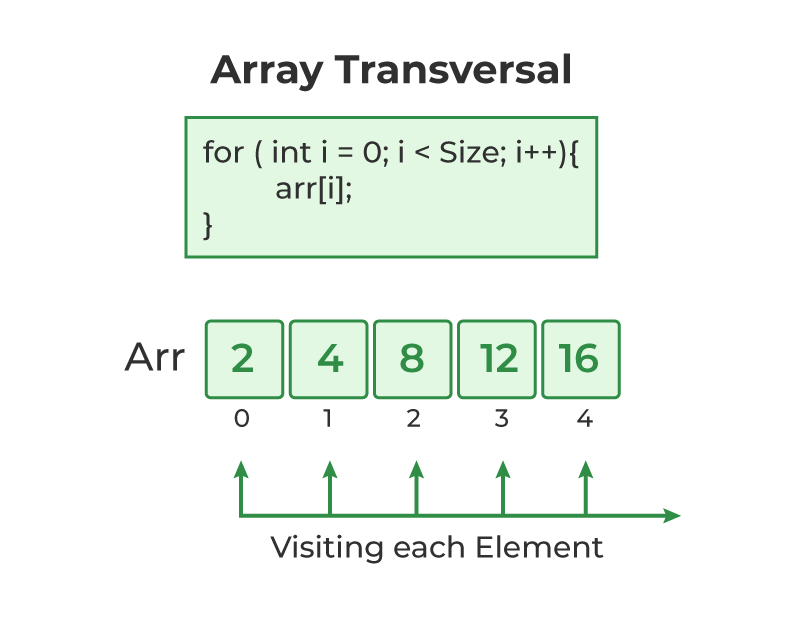## How to use Array in C?

The following program demonstrates how to use an array in the C programming language:

## C

 `// C Program to demonstrate the use of array` `#include `   `int` `main()` `{` `    ``// array declaration and initialization` `    ``int` `arr = { 10, 20, 30, 40, 50 };`   `    ``// modifying element at index 2` `    ``arr = 100;`   `    ``// traversing array using for loop` `  ``printf``(``"Elements in Array: "``);` `    ``for` `(``int` `i = 0; i < 5; i++) {` `        ``printf``(``"%d "``, arr[i]);` `    ``}`   `    ``return` `0;` `}`

Output

`Elements in Array: 10 20 100 40 50 `

## Types of Array in C

There are two types of arrays based on the number of dimensions it has. They are as follows:

1. One Dimensional Arrays (1D Array)
2. Multidimensional Arrays

## 1. One Dimensional Array in C

The One-dimensional arrays, also known as 1-D arrays in C are those arrays that have only one dimension.

### Syntax of 1D Array in C

`array_name [size];`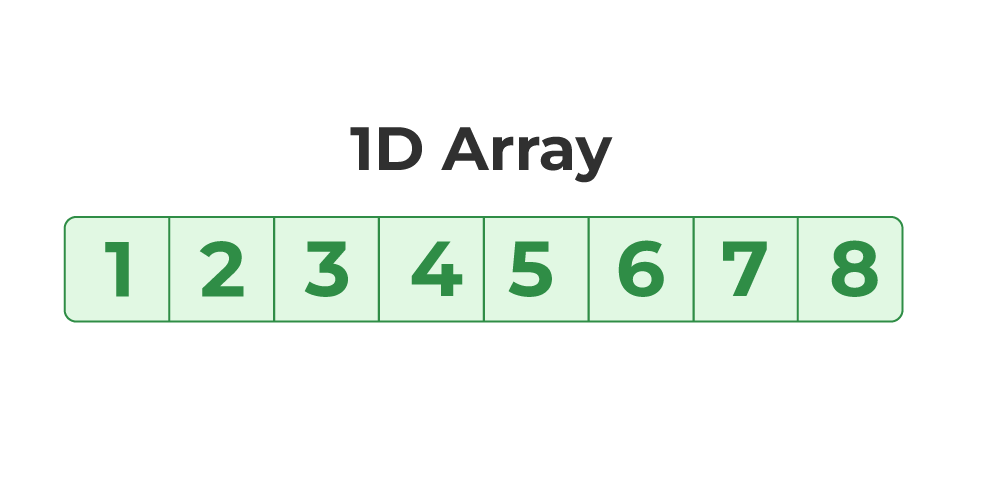## C

 `// C Program to illustrate the use of 1D array` `#include `   `int` `main()` `{`   `    ``// 1d array declaration` `    ``int` `arr;`   `    ``// 1d array initialization using for loop` `    ``for` `(``int` `i = 0; i < 5; i++) {` `        ``arr[i] = i * i - 2 * i + 1;` `    ``}`   `    ``printf``(``"Elements of Array: "``);` `    ``// printing 1d array by traversing using for loop` `    ``for` `(``int` `i = 0; i < 5; i++) {` `        ``printf``(``"%d "``, arr[i]);` `    ``}`   `    ``return` `0;` `}`

Output

`Elements of Array: 1 0 1 4 9 `

### Array of Characters (Strings)

In C, we store the words, i.e., a sequence of characters in the form of an array of characters terminated by a NULL character. These are called strings in C language.

## C

 `// C Program to illustrate strings` `#include `   `int` `main()` `{`   `    ``// creating array of character` `    ``char` `arr = { ``'G'``, ``'e'``, ``'e'``, ``'k'``, ``'s'``, ``'\0'` `};`   `    ``// printing string` `    ``int` `i = 0;` `    ``while` `(arr[i]) {` `        ``printf``(``"%c"``, arr[i++]);` `    ``}` `    ``return` `0;` `}`

Output

`Geeks`

## 2. Multidimensional Array in C

Multi-dimensional Arrays in C are those arrays that have more than one dimension. Some of the popular multidimensional arrays are 2D arrays and 3D arrays. We can declare arrays with more dimensions than 3d arrays but they are avoided as they get very complex and occupy a large amount of space.

### A. Two-Dimensional Array in C

A Two-Dimensional array or 2D array in C is an array that has exactly two dimensions. They can be visualized in the form of rows and columns organized in a two-dimensional plane.

### Syntax of 2D Array in C

`array_name[size1] [size2];`

Here,

• size1: Size of the first dimension.
• size2: Size of the second dimension.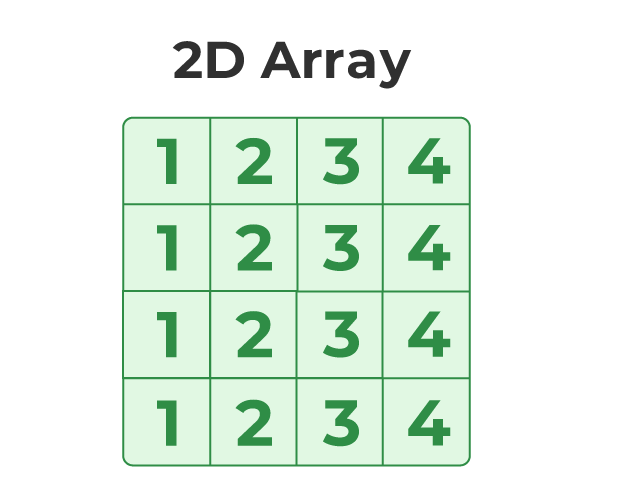## C

 `// C Program to illustrate 2d array` `#include `   `int` `main()` `{`   `    ``// declaring and initializing 2d array` `    ``int` `arr = { 10, 20, 30, 40, 50, 60 };`   `  ``printf``(``"2D Array:\n"``);` `    ``// printing 2d array` `    ``for` `(``int` `i = 0; i < 2; i++) {` `        ``for` `(``int` `j = 0; j < 3; j++) {` `            ``printf``(``"%d "``,arr[i][j]);` `        ``}` `        ``printf``(``"\n"``);` `    ``}`   `    ``return` `0;` `}`

Output

```2D Array:
10 20 30
40 50 60 ```

### B. Three-Dimensional Array in C

Another popular form of a multi-dimensional array is Three Dimensional Array or 3D Array. A 3D array has exactly three dimensions. It can be visualized as a collection of 2D arrays stacked on top of each other to create the third dimension.

### Syntax of 3D Array in C

`array_name [size1] [size2] [size3];`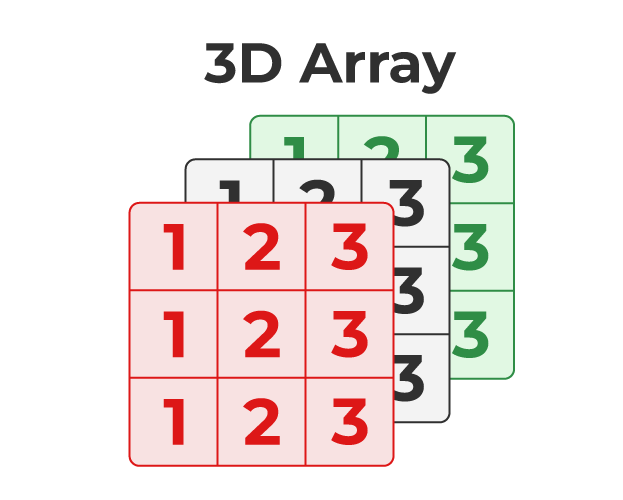## C

 `// C Program to illustrate the 3d array` `#include `   `int` `main()` `{`   `    ``// 3D array declaration` `    ``int` `arr = { 10, 20, 30, 40, 50, 60 };`   `    ``// printing elements` `    ``for` `(``int` `i = 0; i < 2; i++) {` `        ``for` `(``int` `j = 0; j < 2; j++) {` `            ``for` `(``int` `k = 0; k < 2; k++) {` `                ``printf``(``"%d "``, arr[i][j][k]);` `            ``}` `            ``printf``(``"\n"``);` `        ``}` `        ``printf``(``"\n \n"``);` `    ``}` `    ``return` `0;` `}`

Output

```10 20
30 40

50 60
0 0

```

To know more about Multidimensional Array in C, refer to this article – Multidimensional Arrays in C

## Relationship between Arrays and Pointers

Arrays and Pointers are closely related to each other such that we can use pointers to perform all the possible operations of the array. The array name is a constant pointer to the first element of the array and the array decays to the pointers when passed to the function.

## C

 `// C Program to demonstrate the relation between arrays and` `// pointers` `#include `   `int` `main()` `{`   `    ``int` `arr = { 10, 20, 30, 40, 50 };` `    ``int``* ptr = &arr;`   `    ``// comparing address of first element and address stored` `    ``// inside array name` `    ``printf``(``"Address Stored in Array name: %p\nAddress of "` `           ``"1st Array Element: %p\n"``,` `           ``arr, &arr);`   `    ``// printing array elements using pointers` `    ``printf``(``"Array elements using pointer: "``);` `    ``for` `(``int` `i = 0; i < 5; i++) {` `        ``printf``(``"%d "``, *ptr++);` `    ``}` `    ``return` `0;` `}`

Output

```Address Stored in Array name: 0x7ffce72c2660
Address of 1st Array Element: 0x7ffce72c2660
Array elements using pointer: 10 20 30 40 50 ```

To know more about the relationship between an array and a pointer, refer to this article – Pointer to an Arrays | Array Pointer

## Passing an Array to a Function in C

An array is always passed as pointers to a function in C. Whenever we try to pass an array to a function, it decays to the pointer and then passed as a pointer to the first element of an array.

We can verify this using the following C Program:

## C

 `// C Program to pass an array to a function` `#include `   `void` `printArray(``int` `arr[])` `{` `    ``printf``(``"Size of Array in Functions: %d\n"``, ``sizeof``(arr));` `    ``printf``(``"Array Elements: "``);` `    ``for` `(``int` `i = 0; i < 5; i++) {` `        ``printf``(``"%d "``,arr[i]);` `    ``}` `}`   `// driver code` `int` `main()` `{`   `    ``int` `arr = { 10, 20, 30, 40, 50 };`   `    ``printf``(``"Size of Array in main(): %d\n"``, ``sizeof``(arr));` `    ``printArray(arr);` `    ``return` `0;` `}`

Output

```Size of Array in main(): 20
Size of Array in Functions: 8
Array Elements: 10 20 30 40 50 ```

## Return an Array from a Function in C

In C, we can only return a single value from a function. To return multiple values or elements, we have to use pointers. We can return an array from a function using a pointer to the first element of that array.

## C

 `// C Program to return array from a function` `#include `   `// function` `int``* func()` `{` `    ``static` `int` `arr = { 1, 2, 3, 4, 5 };`   `    ``return` `arr;` `}`   `// driver code` `int` `main()` `{`   `    ``int``* ptr = func();`   `    ``printf``(``"Array Elements: "``);` `    ``for` `(``int` `i = 0; i < 5; i++) {` `        ``printf``(``"%d "``, *ptr++);` `    ``}` `    ``return` `0;` `}`

Output

`Array Elements: 1 2 3 4 5 `

Note: You may have noticed that we declared static array using static keyword. This is due to the fact that when a function returns a value, all the local variables and other entities declared inside that function are deleted. So, if we create a local array instead of static, we will get segmentation fault while trying to access the array in the main function.

## Properties of Arrays in C

It is very important to understand the properties of the C array so that we can avoid bugs while using it. The following are the main properties of an array in C:

### 1. Fixed Size

The array in C is a fixed-size collection of elements. The size of the array must be known at the compile time and it cannot be changed once it is declared.

### 2. Homogeneous Collection

We can only store one type of element in an array. There is no restriction on the number of elements but the type of all of these elements must be the same.

### 3. Indexing in Array

The array index always starts with 0 in C language. It means that the index of the first element of the array will be 0 and the last element will be N – 1.

### 4. Dimensions of an Array

A dimension of an array is the number of indexes required to refer to an element in the array. It is the number of directions in which you can grow the array size.

### 5. Contiguous Storage

All the elements in the array are stored continuously one after another in the memory. It is one of the defining properties of the array in C which is also the reason why random access is possible in the array.

### 6. Random Access

The array in C provides random access to its element i.e we can get to a random element at any index of the array in constant time complexity just by using its index number.

### 7. No Index Out of Bounds Checking

There is no index out-of-bounds checking in C/C++, for example, the following program compiles fine but may produce unexpected output when run.

## C

 `// This C program compiles fine` `// as index out of bound` `// is not checked in C.`   `#include `   `int` `main()` `{` `    ``int` `arr;`   `    ``printf``(``"%d "``, arr);` `    ``printf``(``"%d "``, arr[-2]);`   `    ``return` `0;` `}`

Output

`211343841 4195777 `

In C, it is not a compiler error to initialize an array with more elements than the specified size. For example, the below program compiles fine and shows just a Warning.

## C

 `#include ` `int` `main()` `{`   `    ``// Array declaration by initializing it ` `    ``// with more elements than specified size.` `    ``int` `arr = { 10, 20, 30, 40, 50 };`   `    ``return` `0;` `}`

Warnings:

```prog.c: In function 'main':
prog.c:7:25: warning: excess elements in array initializer
int arr = { 10, 20, 30, 40, 50 };
^
prog.c:7:25: note: (near initialization for 'arr')
prog.c:7:29: warning: excess elements in array initializer
int arr = { 10, 20, 30, 40, 50 };
^
prog.c:7:29: note: (near initialization for 'arr')
prog.c:7:33: warning: excess elements in array initializer
int arr = { 10, 20, 30, 40, 50 };
^
prog.c:7:33: note: (near initialization for 'arr')```

## Examples of Array in C

### Example 1: C Program to perform array input and output.

In this program, we will use scanf() and print() function to take input and print output for the array.

## C

 `// C Program to perform input and output on array` `#include `   `int` `main()` `{`   `    ``// declaring an integer array` `    ``int` `arr;`   `    ``// taking input to array elements one by one` `    ``for` `(``int` `i = 0; i < 5; i++) {` `        ``scanf``(``"%d"``, &arr[i]);` `    ``}`   `    ``// printing array elements` `    ``printf``(``"Array Elements: "``);` `    ``for` `(``int` `i = 0; i < 5; i++) {` `        ``printf``(``"%d "``, arr[i]);` `    ``}` `    ``return` `0;` `}`

Input

`5 7 9 1 4`

Output

`Array Elements: 5 7 9 1 4`

### Example 2: C Program to print the average of the given list of numbers

In this program, we will store the numbers in an array and traverse it to calculate the average of the number stored.

## C

 `// C Program to the average to two numbers` `#include `   `// function to calculate average of the function` `float` `getAverage(``float``* arr, ``int` `size)` `{`   `    ``int` `sum = 0;` `    ``// calculating cumulative sum of all the array elements` `    ``for` `(``int` `i = 0; i < size; i++) {` `        ``sum += arr[i];` `    ``}`   `    ``// returning average` `    ``return` `sum / size;` `}`   `// driver code` `int` `main()` `{`   `    ``// declaring and initializing array` `    ``float` `arr = { 10, 20, 30, 40, 50 };` `    ``// size of array using sizeof operator` `    ``int` `n = ``sizeof``(arr) / ``sizeof``(``float``);`   `    ``// printing array elements` `    ``printf``(``"Array Elements: "``);` `    ``for` `(``int` `i = 0; i < n; i++) {` `        ``printf``(``"%.0f "``, arr[i]);` `    ``}`   `    ``// calling getAverage function and printing average` `    ``printf``(``"\nAverage: %.2f"``, getAverage(arr, n));` `    ``return` `0;` `}`

Output

```Array Elements: 10 20 30 40 50
Average: 30.00```

## C

 `// C Program to find the largest number in the array.` `#include `   `// function to return max value` `int` `getMax(``int``* arr, ``int` `size)` `{` `    ``int` `max = arr;` `    ``for` `(``int` `i = 1; i < size; i++) {` `        ``if` `(max < arr[i]) {` `            ``max = arr[i];` `        ``}` `    ``}` `    ``return` `max;` `}`   `// Driver code` `int` `main()` `{`   `    ``int` `arr` `        ``= { 135, 165, 1, 16, 511, 65, 654, 654, 169, 4 };`   `    ``printf``(``"Largest Number in the Array: %d"``,` `           ``getMax(arr, 10));`   `    ``return` `0;` `}`

Output

`Largest Number in the Array: 654`

## Advantages of Array in C

The following are the main advantages of an array:

1. Random and fast access of elements using the array index.
2. Use of fewer lines of code as it creates a single array of multiple elements.
3. Traversal through the array becomes easy using a single loop.
4. Sorting becomes easy as it can be accomplished by writing fewer lines of code.

## Disadvantages of Array in C

1. Allows a fixed number of elements to be entered which is decided at the time of declaration. Unlike a linked list, an array in C is not dynamic.
2. Insertion and deletion of elements can be costly since the elements are needed to be rearranged after insertion and deletion.

## Conclusion

The array is one of the most used and important data structures in C. It is one of the core concepts of C language that is used in every other program. Though it is important to know about its limitation so that we can take advantage of its functionality.

## FAQs on C Array

### 1. Define Array in C.

An array is a fixed-size homogeneous collection of elements that are stored in a contiguous memory location.

### 2. How to declare an array in C?

We can declare array in C using the following syntax:

`datatype array_name [size];`

### 3. How do you initialize an array in C?

We can initialize an array using two methods:

• Using Initializer list
• Using Loops

#### Using Initializer List

We can use an initializer list to initialize the array with the declaration using the following syntax:

`datatype array_name [size] = {value1, value2,...valueN};`

#### Using Loops

We can initialize an Array using Loops in C after the array declaration:

```for (int i = 0; i < N; i++) {
array_name[i] = valuei;
}```

### 4. Why do we need Arrays?

We can use normal variables (v1, v2, v3, ..) when we have a small number of objects, but if we want to store a large number of instances, it becomes difficult to manage them with normal variables. The idea of an array is to represent many instances in one variable.

### 5. How can we determine the size of the C array?

We can determine the size of the Array using sizeof Operator in C. We first get the size of the whole array and divide it by the size of each element type.

### 6. What is the difference between Arrays and Pointers?

The following table list the differences between an array and a pointer:

My Personal Notes arrow_drop_up